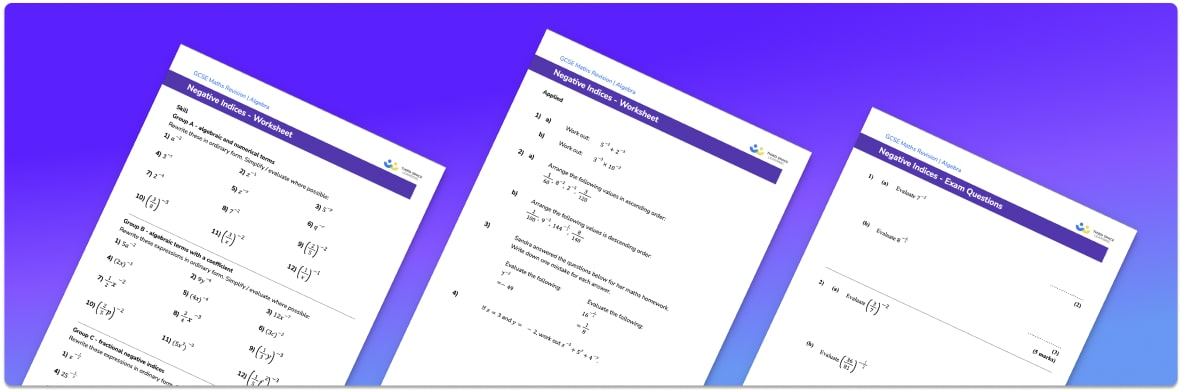# Negative Indices Worksheet• Section 1 of the negative indices worksheet contains 20+ skills-based negative indices questions, in 3 groups to support differentiation
• Section 2 contains 3 applied negative indices questions with a mix of worded problems and deeper problem solving questions
• Section 3 contains 3 foundation and higher level GCSE exam questions on negative indices
• Answers and a mark scheme for all negative indices questions are provided
• Questions follow variation theory with plenty of opportunities for students to work independently at their own level
• All questions created by fully qualified expert secondary maths teachers
• Suitable for GCSE maths revision for AQA, OCR and Edexcel exam boards

• This field is for validation purposes and should be left unchanged.

You can unsubscribe at any time (each email we send will contain an easy way to unsubscribe). To find out more about how we use your data, see our privacy policy.

### Negative indices at a glance

Negative indices are powers, or exponents, which are negative. To deal with negative powers, we find the reciprocal of the term. We put the term over 1 and then ‘flip’ the fraction. Doing this changes the sign of the power, making it a positive index on the denominator of the fraction.

We can also have negative fractional powers. For fractional powers, the denominator indicates the root of the number, which is then raised to the power of the numerator. For example the power of 1/2 means find the square root and the power of 2/3  means calculate the cube root and then square the answer.

The rules of indices can be applied to expressions involving negative indices as well as expressions where the powers are positive integers, decimals or fractions.

Looking forward, students can then progress to additional algebra worksheets, for example a simplifying expressions worksheet or simultaneous equations worksheet.For more teaching and learning support on Algebra our GCSE maths lessons provide step by step support for all GCSE and IGCSE maths concepts.

## Do you have KS4 students who need more focused attention to succeed at GCSE?There will be students in your class who require individual attention to help them succeed in their maths GCSEs. In a class of 30, it’s not always easy to provide.

Help your students feel confident with exam-style questions and the strategies they’ll need to answer them correctly with our dedicated GCSE maths revision programme.

Lessons are selected to provide support where each student needs it most, and specially-trained GCSE maths tutors adapt the pitch and pace of each lesson. This ensures a personalised revision programme that raises grades and boosts confidence.

Find out more